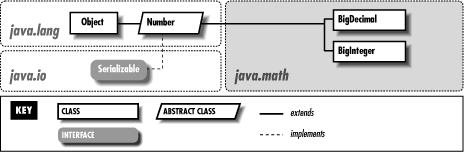home | O'Reilly's CD bookshelfs | FreeBSD | Linux | Cisco | Cisco Exam

# 27. The java.math Package

Contents:
java.math.BigDecimal (JDK 1.1)
java.math.BigInteger (JDK 1.1)

The java.math package, new in Java 1.1, contains classes for arbitrary-precision integer and floating-point arithmetic. Arbitrary-length integers are required for cryptography, and arbitrary-precision floating-point values are useful for financial applications that need to be careful about rounding errors. The class hierarchy of this extremely small package is shown in Figure 27.1.

#### Figure 27.1: The java.math package## 27.1 java.math.BigDecimal (JDK 1.1)

This subclass of java.lang.Number represents a floating-point number of arbitrary size and precision. Its methods duplicate the functionality of the standard Java arithmetic operators. The compareTo() method compares the value of two BigDecimal objects and returns -1, 0, or 1 to indicate the result of the comparison.

BigDecimal objects are represented as an integer of arbitrary size and an integer scale that specifies the number of decimal places in the value. When working with BigDecimal values, you can explicitly specify the amount of precision (the number of decimal places) you are interested in. Also, whenever a BigDecimal method may discard precision (in a division operation, for example), you are required to specify what sort of rounding should be performed on the digit to the left of the discarded digit or digits. The eight constants defined by this class specify the available rounding modes. Because the BigDecimal class provides arbitrary precision and gives you explicit control over rounding and the number of decimal places you are interested in, it can be useful when dealing with quantities that represent money, or in other circumstances where the tolerance for rounding errors is low.

```public class BigDecimal extends Number {
// Public Constructors
public BigDecimal(String val) throws NumberFormatException;
public BigDecimal(double val) throws NumberFormatException;
public BigDecimal(BigInteger val);
public BigDecimal(BigInteger val, int scale) throws NumberFormatException;
// Constants
public static final int ROUND_CEILING;
public static final int ROUND_DOWN;
public static final int ROUND_FLOOR;
public static final int ROUND_HALF_DOWN;
public static final int ROUND_HALF_EVEN;
public static final int ROUND_HALF_UP;
public static final int ROUND_UNNECESSARY;
public static final int ROUND_UP;
// Class Methods
public static BigDecimal valueOf(long val, int scale) throws NumberFormatException;
public static BigDecimal valueOf(long val);
// Public Instance Methods
public BigDecimal abs();
public int compareTo(BigDecimal val);
public BigDecimal divide(BigDecimal val, int scale, int roundingMode) throws ArithmeticException, IllegalArgumentException;
public BigDecimal divide(BigDecimal val, int roundingMode) throws ArithmeticException, IllegalArgumentException;
public double doubleValue();  // Defines Number
public boolean equals(Object x);  // Overrides Object
public float floatValue();  // Defines Number
public int hashCode();  // Overrides Object
public int intValue();  // Defines Number
public long longValue();  // Defines Number
public BigDecimal max(BigDecimal val);
public BigDecimal min(BigDecimal val);
public BigDecimal movePointLeft(int n);
public BigDecimal movePointRight(int n);
public BigDecimal multiply(BigDecimal val);
public BigDecimal negate();
public int scale();
public BigDecimal setScale(int scale, int roundingMode) throws ArithmeticException, IllegalArgumentException;
public BigDecimal setScale(int scale) throws ArithmeticException, IllegalArgumentException;
public int signum();
public BigDecimal subtract(BigDecimal val);
public BigInteger toBigInteger();
public String toString();  // Overrides Object
}
```

### Hierarchy:

Object->Number(Serializable)->BigDecimaljava.lang.reflect.Modifier (JDK 1.1)java.math.BigInteger (JDK 1.1)